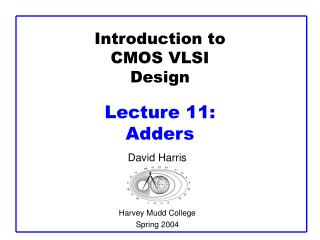# Introduction to CMOS VLSI Design Lecture 11: Adders - PowerPoint PPT PresentationDownload PresentationIntroduction to CMOS VLSI Design Lecture 11: Adders

Introduction to CMOS VLSI Design Lecture 11: AddersDownload Presentation## Introduction to CMOS VLSI Design Lecture 11: Adders

- - - - - - - - - - - - - - - - - - - - - - - - - - - E N D - - - - - - - - - - - - - - - - - - - - - - - - - - -
##### Presentation Transcript

1. Introduction toCMOS VLSIDesignLecture 11: Adders David Harris Harvey Mudd College Spring 2004

5. PGK • For a full adder, define what happens to carries • Generate: Cout = 1 independent of C • G = • Propagate: Cout = C • P = • Kill: Cout = 0 independent of C • K = 11: Adders

6. PGK • For a full adder, define what happens to carries • Generate: Cout = 1 independent of C • G = A • B • Propagate: Cout = C • P = A  B • Kill: Cout = 0 independent of C • K = ~A • ~B 11: Adders

7. Full Adder Design I • Brute force implementation from eqns 11: Adders

8. Full Adder Design II • Factor S in terms of Cout S = ABC + (A + B + C)(~Cout) • Critical path is usually C to Cout in ripple adder 11: Adders

9. Layout • Clever layout circumvents usual line of diffusion • Use wide transistors on critical path • Eliminate output inverters 11: Adders

10. Full Adder Design III • Complementary Pass Transistor Logic (CPL) • Slightly faster, but more area 11: Adders

11. Full Adder Design IV • Dual-rail domino • Very fast, but large and power hungry • Used in very fast multipliers 11: Adders

12. Carry Propagate Adders • N-bit adder called CPA • Each sum bit depends on all previous carries • How do we compute all these carries quickly? 11: Adders

13. Carry-Ripple Adder • Simplest design: cascade full adders • Critical path goes from Cin to Cout • Design full adder to have fast carry delay 11: Adders

14. Inversions • Critical path passes through majority gate • Built from minority + inverter • Eliminate inverter and use inverting full adder 11: Adders

15. Generate / Propagate • Equations often factored into G and P • Generate and propagate for groups spanning i:j • Base case • Sum: 11: Adders

16. Generate / Propagate • Equations often factored into G and P • Generate and propagate for groups spanning i:j • Base case • Sum: 11: Adders

19. Carry-Ripple PG Diagram 11: Adders

20. Carry-Ripple PG Diagram 11: Adders

21. PG Diagram Notation 11: Adders

22. Carry-Skip Adder • Carry-ripple is slow through all N stages • Carry-skip allows carry to skip over groups of n bits • Decision based on n-bit propagate signal 11: Adders

23. Carry-Skip PG Diagram For k n-bit groups (N = nk) 11: Adders

24. Carry-Skip PG Diagram For k n-bit groups (N = nk) 11: Adders

25. Variable Group Size Delay grows as O(sqrt(N)) 11: Adders

27. CLA PG Diagram 11: Adders

29. Carry-Select Adder • Trick for critical paths dependent on late input X • Precompute two possible outputs for X = 0, 1 • Select proper output when X arrives • Carry-select adder precomputes n-bit sums • For both possible carries into n-bit group 11: Adders

30. Carry-Increment Adder • Factor initial PG and final XOR out of carry-select 11: Adders

31. Carry-Increment Adder • Factor initial PG and final XOR out of carry-select 11: Adders

32. Variable Group Size • Also buffer noncritical signals 11: Adders

37. Tree Adder Taxonomy • Ideal N-bit tree adder would have • L = log N logic levels • Fanout never exceeding 2 • No more than one wiring track between levels • Describe adder with 3-D taxonomy (l, f, t) • Logic levels: L + l • Fanout: 2f + 1 • Wiring tracks: 2t • Known tree adders sit on plane defined by l + f + t = L-1 11: Adders